# Scientific Notation Scientific Notation A convenient way to

• Slides: 31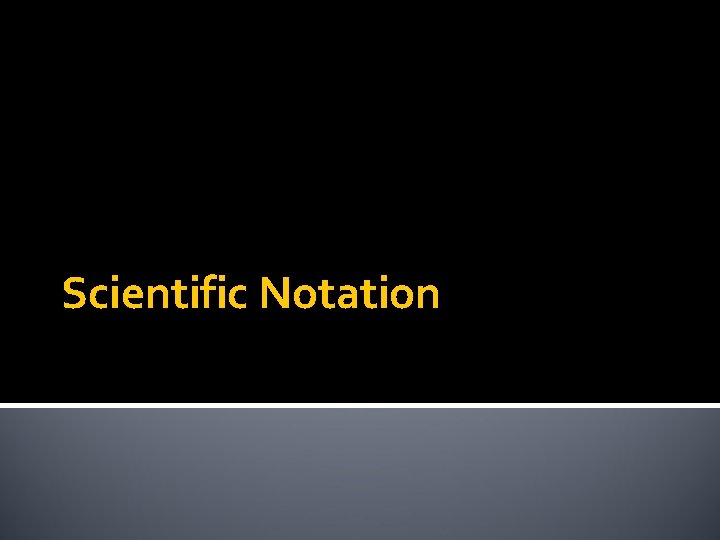Scientific NotationScientific Notation A convenient way to express very large or very small numbers n N x 10 Coefficient Must be 1≤N<10 Exponent (+)—large number (-)—small number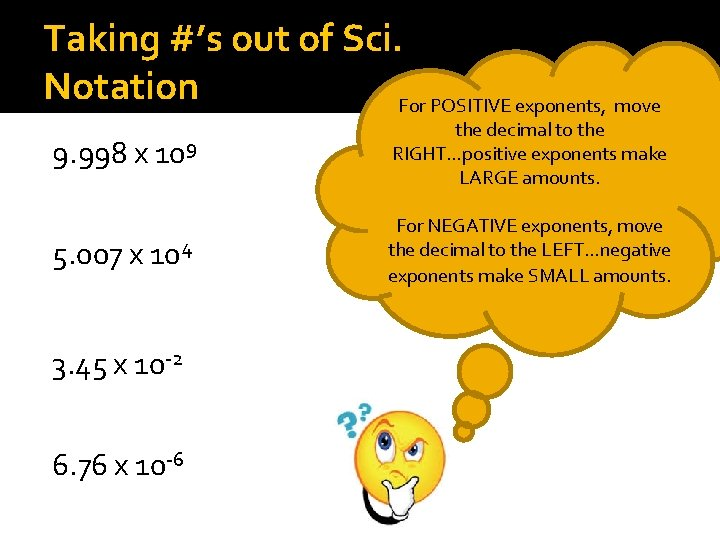Taking #’s out of Sci. Notation For POSITIVE exponents, move 9. 998 x 109 the decimal to the RIGHT…positive exponents make LARGE amounts. 5. 007 x 104 For NEGATIVE exponents, move the decimal to the LEFT…negative exponents make SMALL amounts. 3. 45 x 10 -2 6. 76 x 10 -6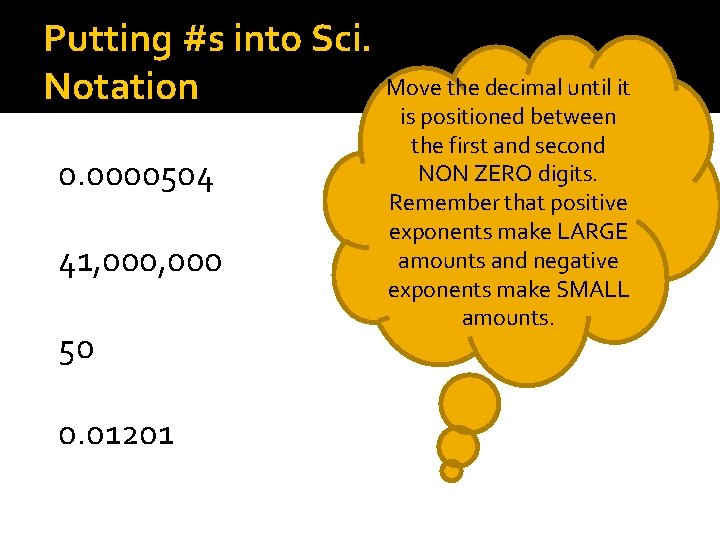Putting #s into Sci. Notation 0. 0000504 41, 000 50 0. 01201 Move the decimal until it is positioned between the first and second NON ZERO digits. Remember that positive exponents make LARGE amounts and negative exponents make SMALL amounts.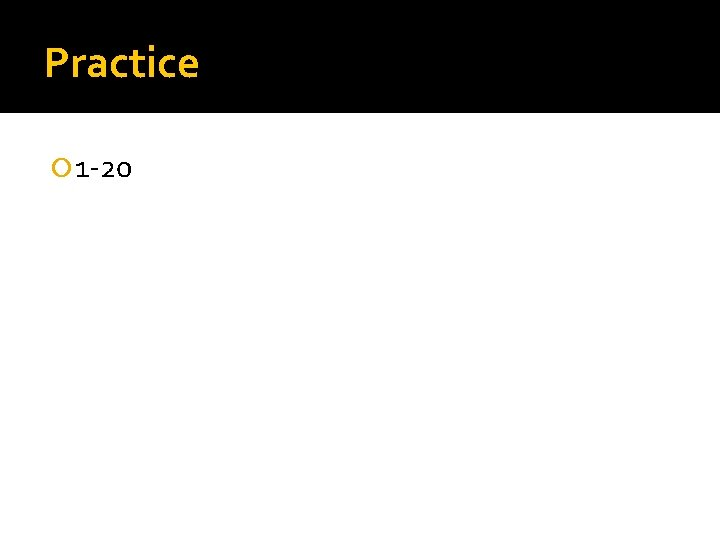Practice 1 -20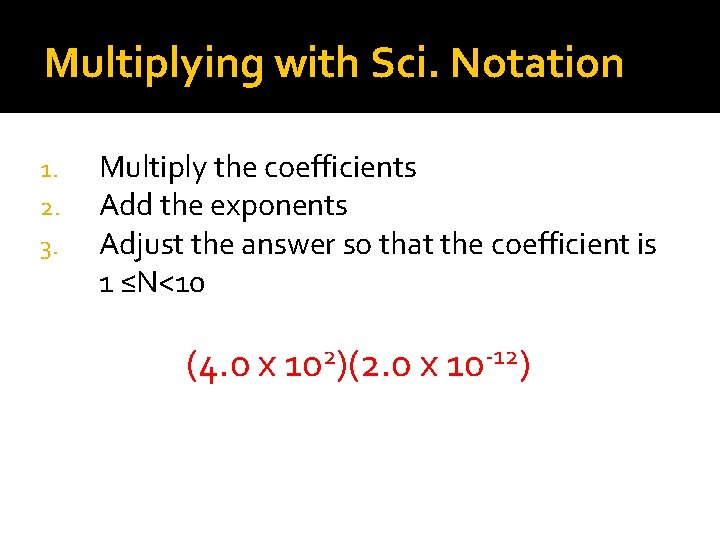Multiplying with Sci. Notation 1. 2. 3. Multiply the coefficients Add the exponents Adjust the answer so that the coefficient is 1 ≤N<10 (4. 0 x 102)(2. 0 x 10 -12)6 -15 (3. 0 x 10 )(8. 0 x 10 )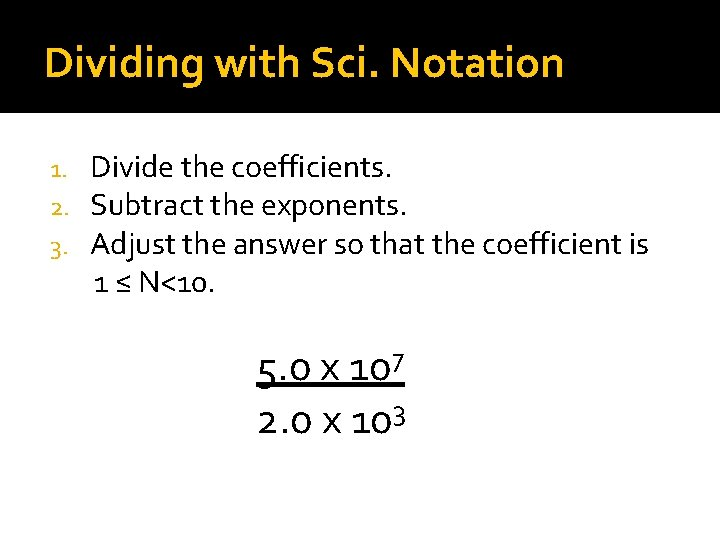Dividing with Sci. Notation Divide the coefficients. Subtract the exponents. Adjust the answer so that the coefficient is 1 ≤ N<10. 1. 2. 3. 7 5. 0 x 10 3 2. 0 x 1012 2. 0 x 10 -3 4. 0 x 10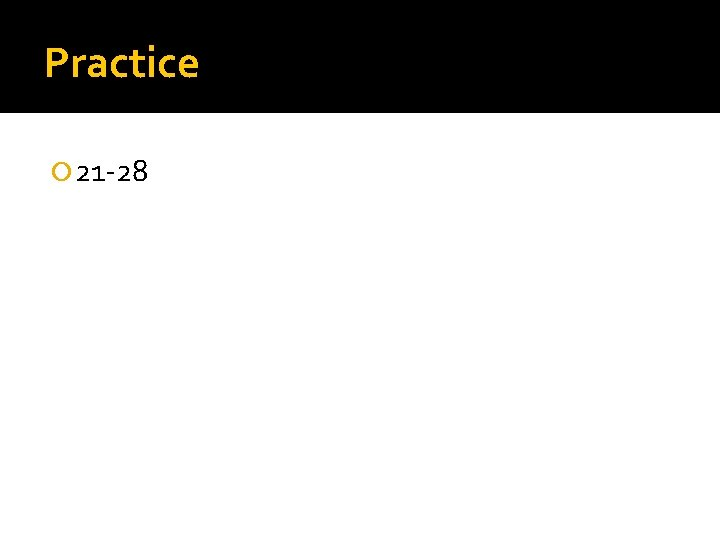Practice 21 -28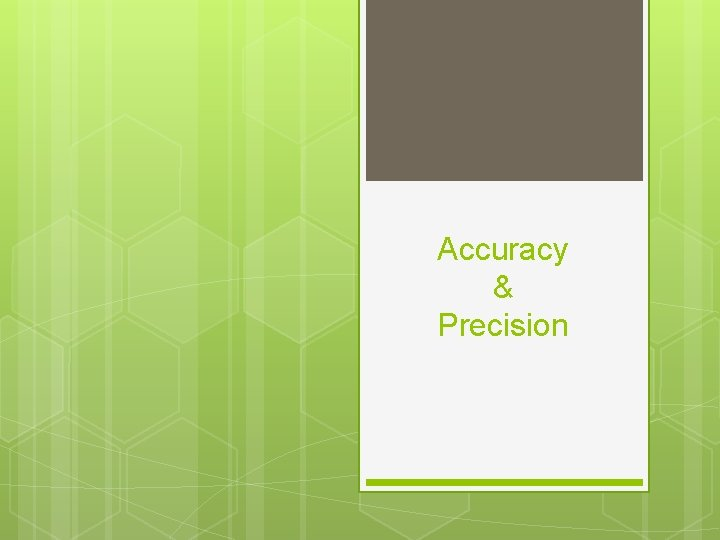Accuracy & Precision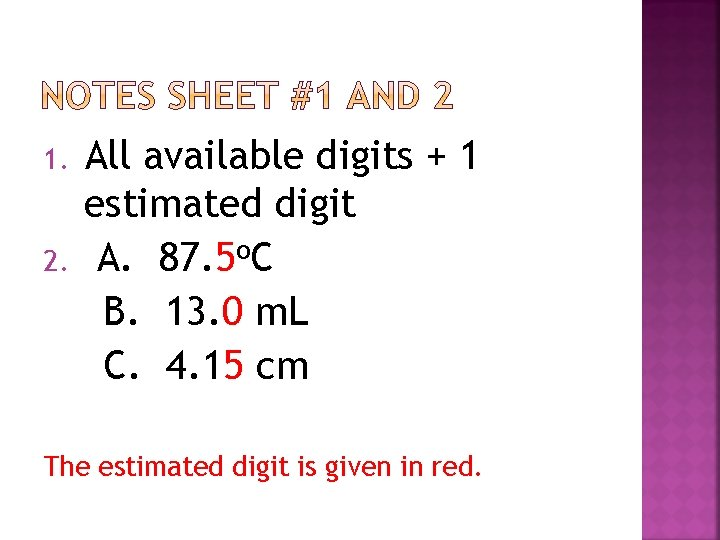1. 2. All available digits + 1 estimated digit A. 87. 5 o. C B. 13. 0 m. L C. 4. 15 cm The estimated digit is given in red.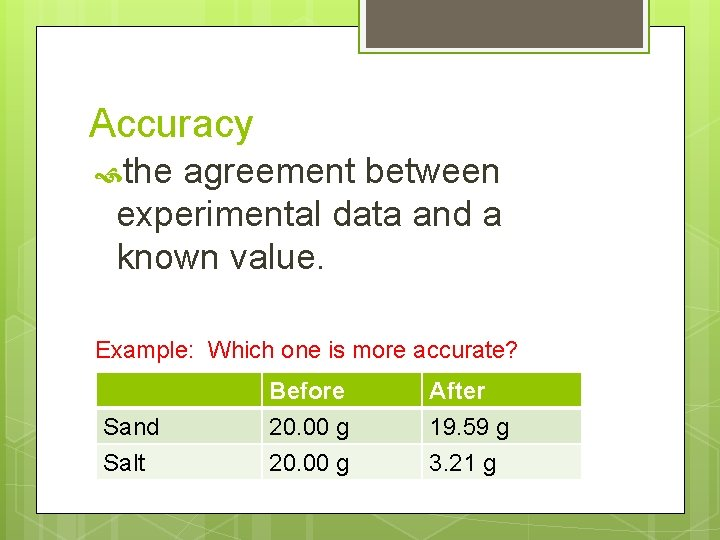Accuracy the agreement between experimental data and a known value. Example: Which one is more accurate? Sand Salt Before 20. 00 g After 19. 59 g 3. 21 g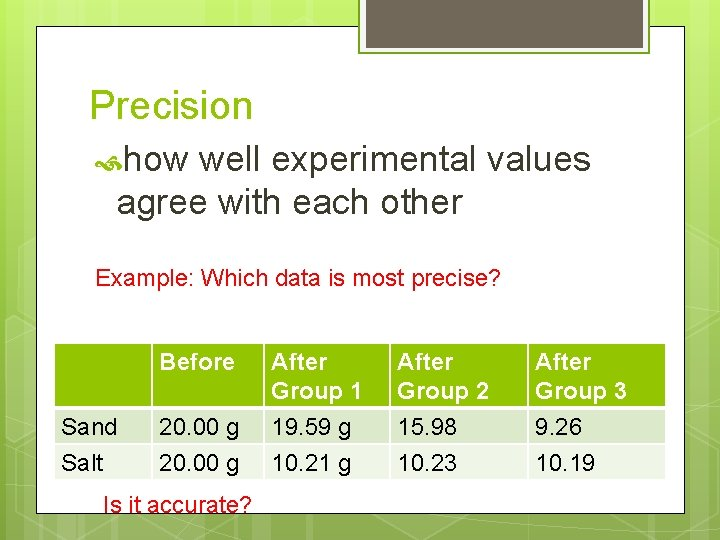Precision how well experimental values agree with each other Example: Which data is most precise? Before Sand Salt 20. 00 g Is it accurate? After Group 1 19. 59 g 10. 21 g After Group 2 15. 98 10. 23 After Group 3 9. 26 10. 19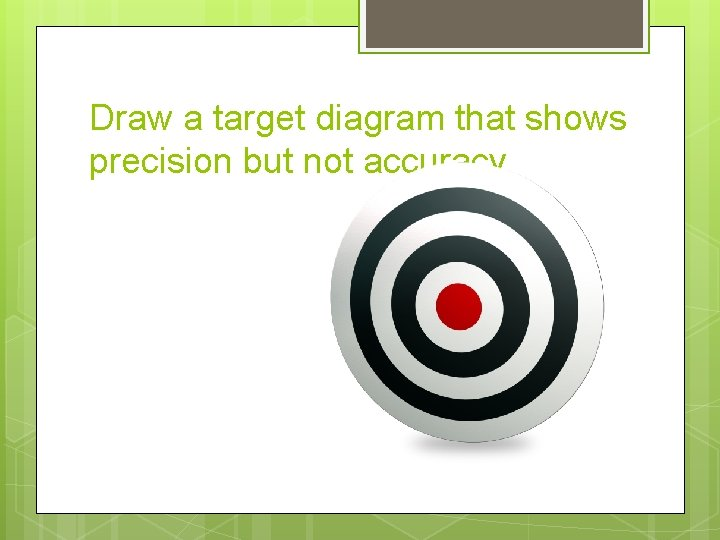Draw a target diagram that shows precision but not accuracy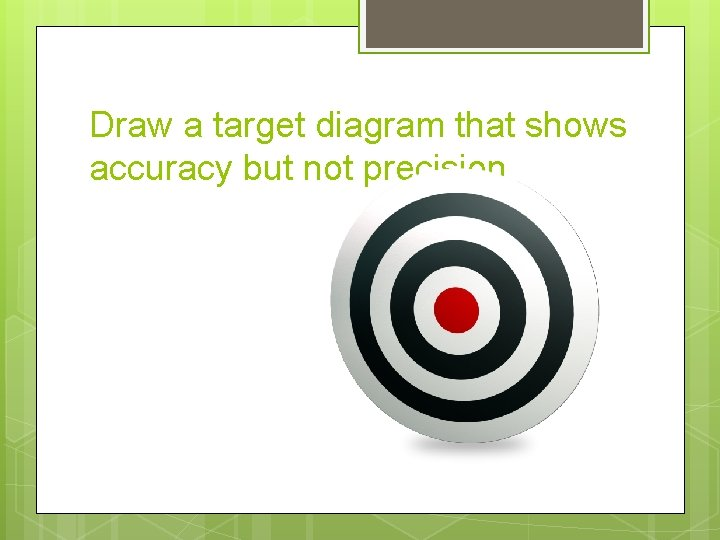Draw a target diagram that shows accuracy but not precisionDraw a target diagram that shows both accuracy and precision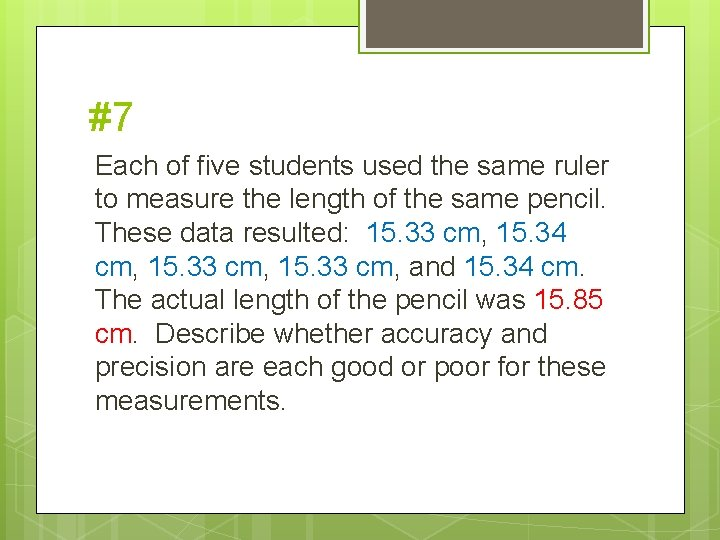#7 Each of five students used the same ruler to measure the length of the same pencil. These data resulted: 15. 33 cm, 15. 34 cm, 15. 33 cm, and 15. 34 cm. The actual length of the pencil was 15. 85 cm. Describe whether accuracy and precision are each good or poor for these measurements.A measurement was taken three times. The correct measurement was 68. 1 m. L. Circle whether the set of measurements is accurate, precise, both, or neither. a) 78. 1 m. L, 43. 9 m. L, 2 m. L accurate b) neither precise both neither 98. 0 m. L, 98. 2 m. L, 97. 9 m. L accurate b) both 68. 1 m. L, 68. 2 m. L, 68. 0 m. L accurate c) precise both neither 72. 0 m. L, 60. 3 m. L, 68. 1 m. L accurate precise both neitherSIGNIFICANT FIGURES PART 1: COUNTING SIG FIGS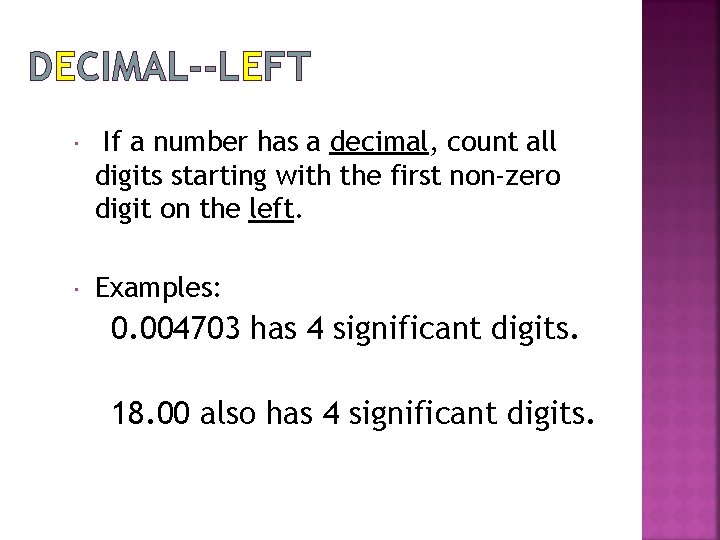DECIMAL--LEFT If a number has a decimal, count all digits starting with the first non-zero digit on the left. Examples: 0. 004703 has 4 significant digits. 18. 00 also has 4 significant digits.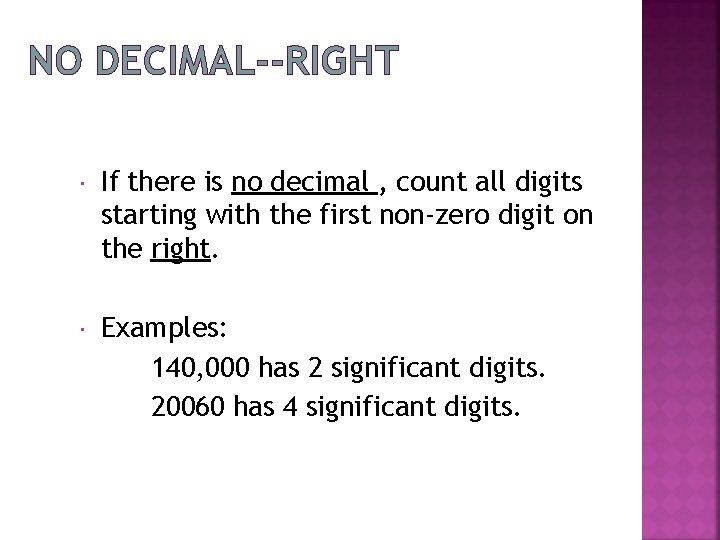NO DECIMAL--RIGHT If there is no decimal , count all digits starting with the first non-zero digit on the right. Examples: 140, 000 has 2 significant digits. 20060 has 4 significant digits.In both cases, start counting with the first non-zero digit. 00. 015 15000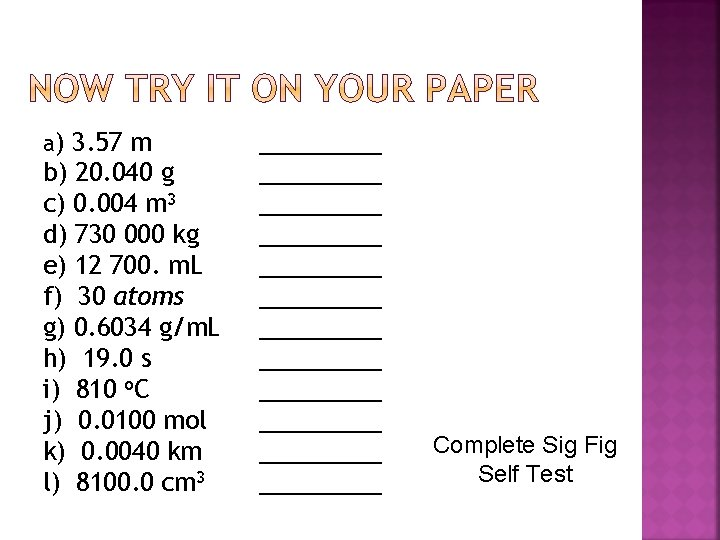a) 3. 57 m b) 20. 040 g c) 0. 004 m 3 d) 730 000 kg e) 12 700. m. L f) 30 atoms g) 0. 6034 g/m. L h) 19. 0 s i) 810 o. C j) 0. 0100 mol k) 0. 0040 km l) 8100. 0 cm 3 _________ _________ _________ Complete Sig Fig Self TestSIGNIFICANT FIGURES PART 2: MATH WITH SIG FIGS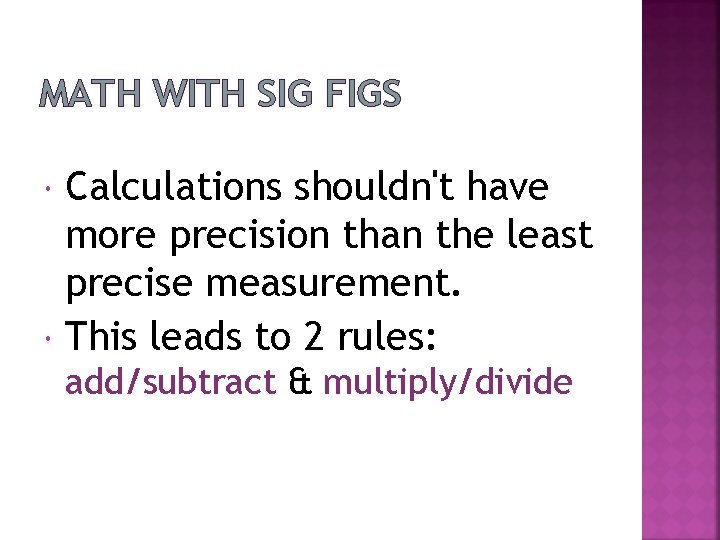MATH WITH SIG FIGS Calculations shouldn't have more precision than the least precise measurement. This leads to 2 rules: add/subtract & multiply/divideADDITION AND SUBTRACTION The answer should not have more decimal places than the number with the least decimal places. Example: 1. 2 + 12. 348 = 4. 2 + 8. 579 =A) 345. 6 + 456. 78 = B) 4. 42 + 8. 576 = C) 23. 456 + 0. 04 = D) 78. 2 - 40 = E) 87. 9 – 20 = F) 478. 84 – 119 =Top half of practice sheet (Addition and Subtraction sets only)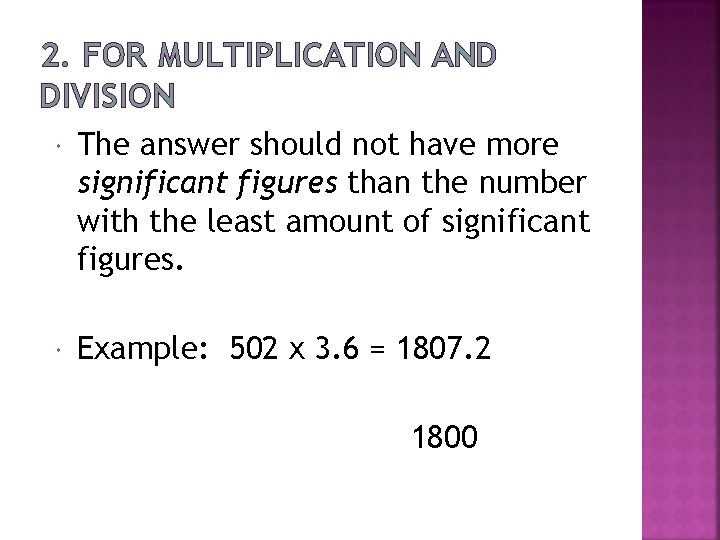2. FOR MULTIPLICATION AND DIVISION The answer should not have more significant figures than the number with the least amount of significant figures. Example: 502 x 3. 6 = 1807. 2 1800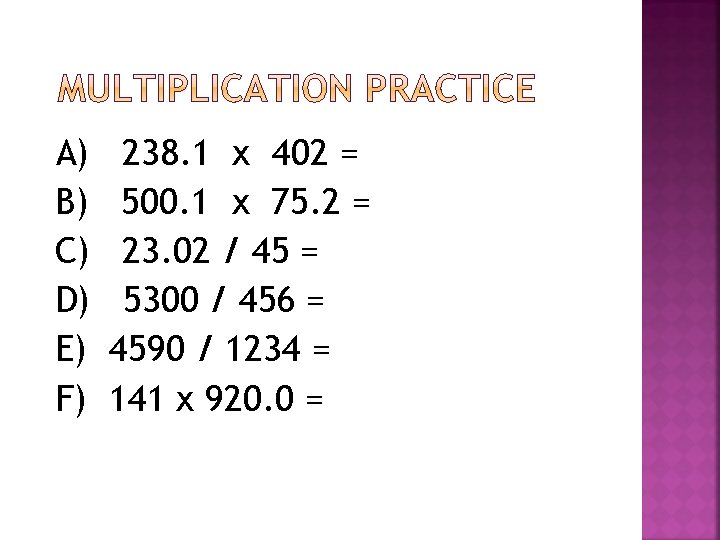A) B) C) D) E) F) 238. 1 x 402 = 500. 1 x 75. 2 = 23. 02 / 45 = 5300 / 456 = 4590 / 1234 = 141 x 920. 0 =